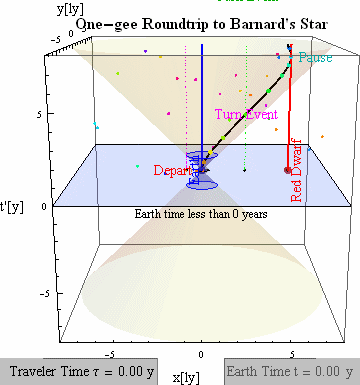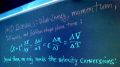# Accel-1D x-ct Plots: Comparing Frames

The animations below show two different perspectives (earth view left, traveler view right) on the same "1-gee" (α~1[ly/y2]) round trip to a star 5.5 lightyears away. Note that the trip (by a little gray rocket with orange exhaust) takes less time from the traveler's perspective, and that when traveling at high speed the traveler and the earth have very different (but self-consistent) ideas about what things happen at the same time. For example in the earth's time slice at left, elapsed time on earth clocks is (c/α)Sinh[ατ/c] = Sinh = 3.63 years when the ship first turns to decelerate (after τ = 2 years on ship-board clocks). In the traveler's time-slice at right, however, only Tanh = 0.96 years have elapsed on earth clocks when the traveler makes that first turn. Both agree on practical matters, like that the first turn-event leaves earth's blue lightcone before 1-Cosh+Sinh = 0.87 earth years have elapsed, and hence that messages to reach the ship before turnabout had better predate that. More on this particular example may be found on our "cool things about vector products" page for students in introductory physics.• For more on the variables here, see this slide, this PDF, or.
• This release dated 31 Mar 1996 (Copyright by Phil Fraundorf 1988-1995)
• At UM-StLouis see also: cme, infophys, physics&astronomy, programs, stei-lab, & wuzzlers.
• Other Theory pages: derivations, slow-example, fast-example&twins, x-tv Plots, 4-vectors, rap.

Note: For a much more recent x-ct application, check out Lorentz from the metric.

One can use a single x-tv plot to solve and visualize all variables in any constant acceleration problem involving only a single traveler. However, many of the challenges to our intuition imposed by relativity involve comparing the experience of a common set of events by travelers in two different inertial frames. If we are content visualizing only the relative position and time of events in a relativity problem, x-ct plots allow us to solve and visualize such problems from the vantage point of two or more inertial frames on a single graph!

Here we describe the strategy for solving such problems when they require only one spatial dimension. We provide links to worked out solutions for some popular applications, like the study of time dilation, length contraction, twin-adventure aging, refrigerator magnets, and the pole/barn challenge.

## Preparing for an x-ct Solution

The first step in examining a set of events with an x-ct plot is to decide which inertial frame to begin the plotting with, i.e. which frame will have orthogonal x and ct axes. It's your choice. Here x is the position coordinate for an event in that orthogonal, home, or "unprimed" coordinate system, t actually refers to the map-time of that event, denoted (in rather arcane fashion for this old series of pages) as b to distinguish it from "low-velocity approximation" Galilean chase-plane time (which may also be used to parameterize relativistic motion), and c is the speed of light, here used to convert time axis units into units like those used for the x-axis. These variables can be used to calculate times and velocities in any kinematic (all of which are plotted on our x-tv plots), but these are the only ones graphed on x-ct plots because they represent a set of coordinates which undergo Lorentz transformation in going from one inertial frame to another. As you will see, after the problem has been sketched with one frame's axes orthogonal, it is often still instructive to see it sketched with orthogonal axes for the other frame, even though both plots predict exactly the same experiences for all observers.

```Example: Consider an accelerated-twin adventure -- One twin stays home and the other accelerates for 2 of her years at 1 ly/yr^2 (or 0.96 g), then decelerates for the next two years to a stop, then accelerates in the home direction for two more years, and then decelerates for 2 years to re-unite with her sibling. How will this set of events look to the unaccelerated twin, and from the vantage point of a second inertial frame moving in the starting direction for the traveling twin at a uniform speed of one roddenberry or lightyear per traveler year (i.e. at 0.707 lightyears per inertial year). Let's choose the most natural inertial frame for this problem, that of the unaccelerated twin, as the frame to be shown with orthogonal x-ct axes. ```

The second step is to draw and label the graph's axes. Where possible, it is nice to make the scale of the x and ct axis the same, so that light rays travel along the diagonals of squares in this plot. Try to choose the axis scales so that events of importance to the problem find themselves somewhere in the field of the plot.

``` Example continued: A calculation like that outlined in our relativistic example yields that our traveling twin will be around 5.5 ly away when she heads back home, and that the inertial time b elapsed, in the frame of our unaccelerated twin, is just under 14.5 years. We therefore include in the range of our plot x-values between -5 and 10 ly, and cb-values between 0 and 15 ly (corresponding to unaccelerated twin elapsed times between 0 and 15 years). ```

``
``` ```

The third step is to plot the events of interest in the problem, as labeled points in the x-ct coordinate space of this orthogonal frame. Such events might be things like "blastoff of the space-ship" or "intiate a retro-rocket deceleration". They might also be things like the shot which begins the 2024 Olympic decathalon's 400meter race, or the adjournment of a meeting. By superposing a grid extrapolated for "tick marks" along the axes of this orthogonal frame, near these events, it will be easy by inspection to see when and where things are happening, from the point of view of our "orthogonal" or "unprimed" inertial frame.

``` Here we've now added the worldline of our traveling twin, with boxes marked at yearly intervals in traveler time. Every other box is labeled as a navigational landmark, as specified in the original problem statement. Some of the orthogonal coordinate grid has been removed to minimize clutter on the plot. Note that indeed the trip takes the traveler 8 years while the horizontal grid lines, drawn at 5 year intervals in the unaccelerated twin's inertial frame, show that the inertial twin will be almost 15 years older when the two are re-united. Note also that the inertial frame time for each anniversary of the lauch, as celebrated by the accelerated twin, can be determined by inspection as well. As you can see, traveler time "slows down" most, to our unaccelerated twin, when she is traveling at the highest speeds. ```

``
``` ```

The last step is to superpose on this plot the axes of the other inertial coordinate system of interest. First find it's origin. Then draw x' and ct' axes tilted from the orthogonal frame axis by an angle theta = arctan(w/c), where w is the relative inertial velocity between the primed and unprimed frames. If the primed frame is moving to the right, these axes will close in toward each other in the upper-right and lower left quadrants of the plot. If the primed frame is moving to the left, relative to the unprimed one, these axes will instead close toward each other in the upper-left and lower-right quadrants of the plot. Tick marks denoting space-time units along the primed coordinate axes will be spaced by an interval which is a factor of Sqrt[1+(w/c)^2]/Sqrt[1-(w/c)^2] larger than the corresponding interval for unprimed frame ticks. Draw these tick marks in with a different style or color than the unprimed frame tick marks, keeping the lines parallel to primed frame rather than unprimed frame axes. As shown below, the "grid" associated with these tick marks is not orthogonal! It may therefore take some practice in learning how to measure with it. Sketch in pieces of this non-orthogonal grid in the neighborhood of the labeled events, so that one can see by inspection when and where the various events are happening from the point of view of our primed observer.

``` Now consider the coordinate axes and grid for a traveler moving to the right with a velocity of 1 roddenberry (i.e. 1 lightyear per traveler year), or 0.707 lightyears per inertial year. The axis angle on this plot is arctan(0.707)=35.2 degrees, while the relative tick mark spacing is Sqrt[3/2]/Sqrt[1/2] = Sqrt = 1.732. Axes and a coordinate grid drawn to this specification are superposed in blue below. It allows you to determine the time & place of any event, from the point of view of this rightward moving system as well. As mentioned above, our accelerated twin's "8 year trip" takes just under 15 years to those in the inertial frame of the unaccelerated twin. Counting the slanted blue tick marks, spaced by 5 years in the rightward moving frame's inertial time, shows that the launch and re-unite events are separated by more than 20 years from that point of view! ```

``
``` ```

``` Had we chosen the rightward moving frame as the one with orthogonal axes, the graph would have looked quite different, as shown below. A careful examination of event coordinates in both systems will show that the two graphs provide exactly the same information for each of the points of view! It just looks different on the plot. ```

``
``` ```

## Example Problems under Construction...

• Time Dilation: Examining the fruit is much better than talking about it.
• Length Contraction: Would you believe 3 separate events involved here?
• Twin-Adventures: See above, and our fast-example&twin page.
• The Pole/Barn Challenge, and the Relativistic Squeeze.
Send ideas/ comments/ questions/ complaints, and register for \ updates to these pages with an email subject-field containing "accel1", to staff@newton.umsl.edu. This page might contain original material. Hence if you echo, in print or on the web, a citation would be cool. `{Thanks. :) /pf}` Mindquilts site page requests ~2000/day approaching a million per year. Requests for a "stat-counter linked subset of pages" since 4/7/2005: .